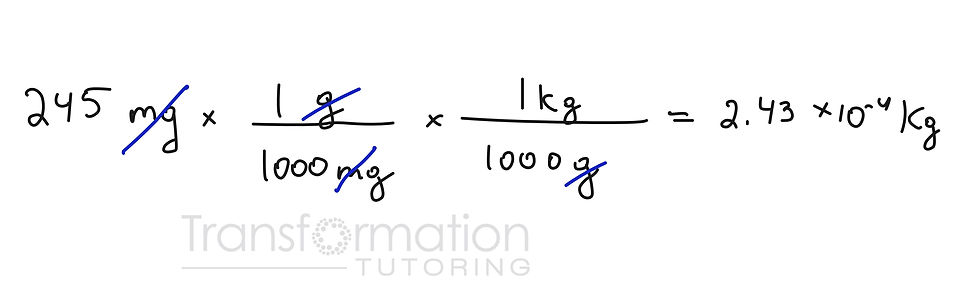top of page
Search

# Unit Conversions (Dimensional Analysis): Complete Guide With Examples

Steps to unit conversions

2. Identify the conversion factor needed to get to the desired unit.

3. Put your units in such a way as to cancel what you have and get the unit you want.

Below, you will find some of the most common prefixes used in unit conversions.unit conversion chart

Let's take a look:

Convert 243 mg into gThe unit we are given are milligrams and we need to cover them to grams. We start with 245 milligrams and to cancel this unit, we need to put it in the denominator and the unit we want (grams) needs to go to the numerator. Who is bigger milligram or gram? Gram! How many milligrams are in 1 gram? 1000

And there you have it!

Sometimes multiple conversions are needed, which can be done in the same step.

Convert 243 mg to kgWe do not know the conversion factor for going from milligrams to kilograms but we do know how to convert milligrams to grams and grams to kilograms!

Volume and Area conversions

Now we know how to convert simple units but what about area and volume where we see squares and cubes?

Convert as you would with simple units. Once you write out the conversion factor, take it into parenthesis and square or cube it depending on the units needed.We start with 25 meters square and 1 meter has 100 cm. We square this conversion factor to get to the right units.

Conversions with numerator and denominator

Sometimes we might have to convert both the numerator and the denominator.

Let's say we want to convert 22 m/hr into km/minWe can first choose the unit we want to convert, either meters or hours. I am doing meters first, and putting my meter conversion first. 1 kilometer has 1000 meters. Meters cancel leaving kilometers. Next, lets convert hours. Hours are in the denominator, to get rid of them we need to put hours in the numerator for the conversion factor and minutes in the denominator. 1 hour has 60 minutes. My hours cancel as well leaving me with minutes. The final result will have km/min unit.

Tags: